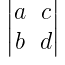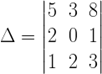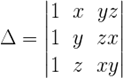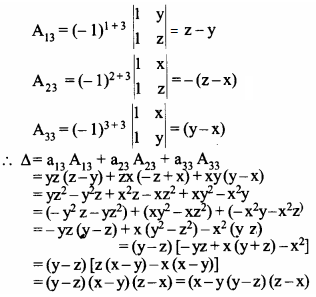NCERT Solutions for Maths Class 12 Exercise 4.4

# NCERT Solutions for Maths Class 12 Exercise 4.4

## NCERT Solutions for Maths Class 12 Exercise 4.4

### Maths Class 12 Ex 4.4 Question 1.

Write the Minors and Cofactors of the elements of following determinants:

(i) Let A =M11 = Minor of a11 = 3, M12 = Minor of a12 = 0,

M21 = Minor of a21 = –4, M22 = Minor of a22 = 2

Now, cofactors of aij is Aij.

So, A11 = (–1)1 + 1 M11 = (–1)2 (3) = 3

A12 = (–1)1 + 2 M12 = (–1)3 (0) = 0

A21 = (–1)2 + 1 M21 = (–1)3 (–4) = 4

A22 = (–1)2 + 2 M22 = (–1)4 (2) = 2

(ii) Let A =M11 = Minor of a11 = d, M12 = Minor of a12 = b,

M21 = Minor of a21 = c, M22 = Minor of a22 = a

Now, cofactors of aij is Aij.

So, A11 = (–1)1 + 1 M11 = (–1)2 (d) = d

A12 = (–1)1 + 2 M12 = (–1)3 (b) = –b

A21 = (–1)2 + 1 M21 = (–1)3 (c) = –c

A22 = (–1)2 + 2 M22 = (–1)4 (a) = a

### Maths Class 12 Ex 4.4 Question 2.

Write Minors and Cofactors of the elements of following determinants:

### Maths Class 12 Ex 4.4 Question 3.

Using Cofactors of elements of second row, evaluateSolution:

The elements of second row are 2, 0 and 1.### Maths Class 12 Ex 4.4 Question 4.

Using Cofactors of elements of third column, evaluateSolution:

The elements of third column are yz, zx and xy.### Maths Class 12 Ex 4.4 Question 5.

Ifand Aij is Cofactors of aij, then value of ∆ is given by
(A) a11 A31 + a12 A32 + a13 A33
(B) a11 A11 + a12 A21 + a13 A31
(C) a21 A11 + a22 A12 + a23 A13
(D) a11 A11 + a21 A21 + a31 A31

Solution:
Option (D) is correct.